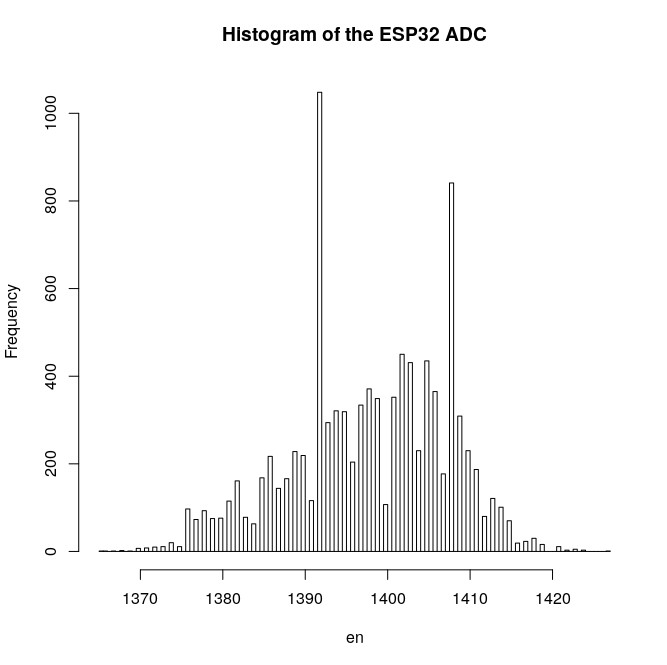ADC: it doesn't read correct values. Problems with conversion.

• Hi everybody! we are using adc pins (P13,P14, P15,P16,P17,P18) to read voltages form a 6 load cells.
The code we used for every cell is:

apin=adc.channel(pin='P18') # for example
val=apin()
print(val)

this code works only for high values and returns the respective values in bits( or analog?) , so we make some tests and we note that it returns 0(bit) when we appply a 73 mV or lower. This is strange because we have 12 bits(4095 levels) so we should appreciate up to 0.01 mv.
how can we solve this problem? maybe do we make some mistakes in the conversion?
Thank you so much !

• @fedesilvi Just to give you an impression how bad the esp32 ADC is, I collected 10000 samples with the ESP32 ADC and an ADS7818 ADC taking values the same LM385 voltage reference. The absolute values differ, because of the different ranges (5V vs. 3.3V). The histograms are below:Edit: Adding a 10 nF capacitor close to the ESP32 ADC input and switching off WiFi improved the picture. The one above was WITH the capacitor. Without, the capacitor, it was worse (avg +/-100 readings)

• @fedesilvi Your observation is right. The ADC does not return values for inputs below 70mV. That's a basic problem of the built-in and other ADC's. If you look at special devices like the HX711, the take their values at an offset with respect to GND.
But anyhow, I wonder why the output of the load cell is close to GND level. I would expect it to be at abouit Vcc/2.
So what you could do is to create a reference in the middle of the voltage range, either with a voltage divider and a capacitor for low dynamic impedance, or an op-amp.
So one side of that load cell bridge would go to that reference point, the other to the ADC input.And don't forget to use one ADC input for taking the value of that reference point. The Load cell output would be the difference between reference and teh respective input.
Besides that, I have doubts that the resolution of the ESP32 ADC is sufficient.